Subscription confirmed. Thank you for subscribing!

# A (Relatively Easy To Understand) Primer on Elliptic Curve Cryptography

Elliptic Curve Cryptography (ECC) is one of the most powerful but least understood types of cryptography in wide use today. At CloudFlare, we make extensive use of ECC to secure everything from our customers' HTTPS connections to how we pass data between our data centers.

Fundamentally, we believe it's important to be able to understand the technology behind any security system in order to trust it. To that end, we looked around to find a good, relatively easy-to-understand primer on ECC in order to share with our users. Finding none, we decided to write one ourselves. That is what follows.

Be warned: this is a complicated subject and it's not possible to boil down to a pithy blog post. In other words, settle in for a bit of an epic because there's a lot to cover. If you just want the gist, the TL;DR is: ECC is the next generation of public key cryptography and, based on currently understood mathematics, provides a significantly more secure foundation than first generation public key cryptography systems like RSA. If you're worried about ensuring the highest level of security while maintaining performance, ECC makes sense to adopt. If you're interested in the details, read on.

### The dawn of public key cryptography

The history of cryptography can be split into two eras: the classical era and the modern era. The turning point between the two occurred in 1977, when both the RSA algorithm and the Diffie-Hellman key exchange algorithm were introduced. These new algorithms were revolutionary because they represented the first viable cryptographic schemes where security was based on the theory of numbers; it was the first to enable secure communication between two parties without a shared secret. Cryptography went from being about securely transporting secret codebooks around the world to being able to have provably secure communication between any two parties without worrying about someone listening in on the key exchange.Whitfield Diffie and Martin Hellman

Modern cryptography is founded on the idea that the key that you use to encrypt your data can be made public while the key that is used to to decrypt your data can be kept private. As such, these systems are known as public key cryptographic systems. The first, and still most widely used of these systems, is known as RSA — named after the initials of the three men who first publicly described the algorithm: Ron Rivest, Adi Shamir and Leonard Adleman.

What you need for a public key cryptographic system to work is a set of algorithms that is easy to process in one direction, but difficult to undo. In the case of RSA, the easy algorithm multiplies two prime numbers. If multiplication is the easy algorithm, its difficult pair algorithm is factoring the product of the multiplication into its two component primes. Algorithms that have this characteristic — easy in one direction, hard the other — are known as Trap door Functions. Finding a good Trapdoor Function is critical to making a secure public key cryptographic system. Simplistically: the bigger the spread between the difficulty of going one direction in a Trapdoor Function and going the other, the more secure a cryptographic system based on it will be.

### A toy RSA algorithm

The RSA algorithm is the most popular and best understood public key cryptography system. Its security relies on the fact that factoring is slow and multiplication is fast. What follows is a quick walk-through of what a small RSA system looks like and how it works.

In general, a public key encryption system has two components, a public key and a private key. Encryption works by taking a message and applying a mathematical operation to it to get a random-looking number. Decryption takes the random looking number and applies a different operation to get back to the original number. Encryption with the public key can only be undone by decrypting with the private key.

Computers don't do well with arbitrarily large numbers. We can make sure that the numbers we are dealing with do not get too large by choosing a maximum number and only dealing with numbers less than the maximum. We can treat the numbers like the numbers on an analog clock. Any calculation that results in a number larger than the maximum gets wrapped around to a number in the valid range.

In RSA, this maximum value (call it max) is obtained by multiplying two random prime numbers. The public and private keys are two specially chosen numbers that are greater than zero and less than the maximum value, call them pub and priv. To encrypt a number you multiply it by itself pub times, making sure to wrap around when you hit the maximum. To decrypt a message, you multiply it by itself priv times and you get back to the original number. It sounds surprising, but it actually works. This property was a big breakthrough when it was discovered.

To create a RSA key pair, first randomly pick the two prime numbers to obtain the maximum (max). Then pick a number to be the public key pub. As long as you know the two prime numbers, you can compute a corresponding private key priv from this public key. This is how factoring relates to breaking RSA — factoring the maximum number into its component primes allows you to compute someone's private key from the public key and decrypt their private messages.

Let's make this more concrete with an example. Take the prime numbers 13 and 7, their product gives us our maximum value of 91. Let's take our public encryption key to be the number 5. Then using the fact that we know 7 and 13 are the factors of 91 and applying an algorithm called the Extended Euclidean Algorithm, we get that the private key is the number 29.

These parameters (max: 91, pub: 5; priv: 29) define a fully functional RSA system. You can take a number and multiply it by itself 5 times to encrypt it, then take that number and multiply it by itself 29 times and you get the original number back.

Let's use these values to encrypt the message "CLOUD".

In order to represent a message mathematically we have to turn the letters into numbers. A common representation of the Latin alphabet is UTF-8. Each character corresponds to a number.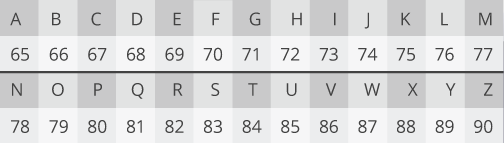Under this encoding, CLOUD is 67, 76, 79, 85, 68. Each of these digits are smaller than our maximum of 91, so we can encrypt them individually. Let's start with the first letter.

We have to multiply it by itself 5 times to get the encrypted value.

67×67 = 4489 = 30 *

*Since 4489 is larger than max, we have to wrap it around. We do that by dividing by 91 and taking the remainder.

4489 = 91×49 + 30

30×67 = 2010 = 8

8×67 = 536 = 81

81×67 = 5427 = 58

This means the encrypted version of 67 is 58.

Repeating the process for each of the letters we get that the encrypted message CLOUD becomes:

58, 20, 53, 50, 87

To decrypt this scrambled message, we take each number and multiply it by itself 29 times:

58×58 = 3364 = 88 (remember, we wrap around when the number is greater than max)>

88×58 = 5104 = 8

9×58 = 522 = 67

Voila, we're back to 67. This works with the rest of the digits, resulting in the original message.

The takeaway is that you can take a number, multiply it by itself a number of times to get a random-looking number, then multiply that number by itself a secret number of times to get back to the original number.

### Not a perfect Trapdoor

RSA and Diffie-Hellman were so powerful because they came with rigorous security proofs. The authors proved that breaking the system is equivalent to solving a mathematical problem that is thought to be difficult to solve. Factoring is a very well known problem and has been studied since antiquity (see Sieve of Eratosthenes). Any breakthroughs would be big news and would net the discoverer a significant financial windfall."Find factors, get money" - Notorious T.K.G. (Reuters)

That said, factoring is not the hardest problem on a bit for bit basis. Specialized algorithms like the Quadratic Sieve and the General Number Field Sieve were created to tackle the problem of prime factorization and have been moderately successful. These algorithms are faster and less computationally intensive than the naive approach of just guessing pairs of known primes.

These factoring algorithms get more efficient as the size of the numbers being factored get larger. The gap between the difficulty of factoring large numbers and multiplying large numbers is shrinking as the number (i.e. the key's bit length) gets larger. As the resources available to decrypt numbers increase, the size of the keys need to grow even faster. This is not a sustainable situation for mobile and low-powered devices that have limited computational power. The gap between factoring and multiplying is not sustainable in the long term.

All this means is that RSA is not the ideal system for the future of cryptography. In an ideal Trapdoor Function, the easy way and the hard way get harder at the same rate with respect to the size of the numbers in question. We need a public key system based on a better Trapdoor.

### Elliptic curves: Building blocks of a better Trapdoor

After the introduction of RSA and Diffie-Hellman, researchers explored other mathematics-based cryptographic solutions looking for other algorithms beyond factoring that would serve as good Trapdoor Functions. In 1985, cryptographic algorithms were proposed based on an esoteric branch of mathematics called elliptic curves.

But what exactly is an elliptic curve and how does the underlying Trapdoor Function work? Unfortunately, unlike factoring — something we all had to do for the first time in middle school — most people aren't as familiar with the math around elliptic curves. The math isn't as simple, nor is explaining it, but I'm going to give it a go over the next few sections. (If your eyes start to glaze over, you can skip way down to the section: What does it all mean.)

An elliptic curve is the set of points that satisfy a specific mathematical equation. The equation for an elliptic curve looks something like this:

y2 = x3 + ax + b

That graphs to something that looks a bit like the Lululemon logo tipped on its side: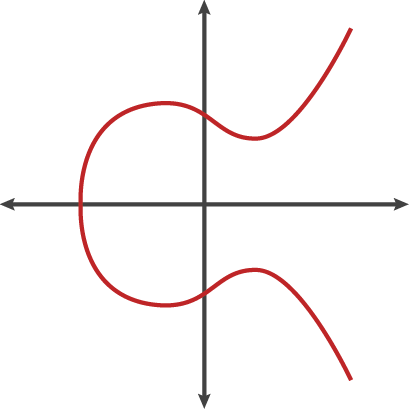There are other representations of elliptic curves, but technically an elliptic curve is the set points satisfying an equation in two variables with degree two in one of the variables and three in the other. An elliptic curve is not just a pretty picture, it also has some properties that make it a good setting for cryptography.

### Strange symmetry

Take a closer look at the elliptic curve plotted above. It has several interesting properties.

One of these is horizontal symmetry. Any point on the curve can be reflected over the x axis and remain the same curve. A more interesting property is that any non-vertical line will intersect the curve in at most three places.

Let's imagine this curve as the setting for a bizarre game of billiards. Take any two points on the curve and draw a line through them, it will intersect the curve at exactly one more place. In this game of billiards, you take a ball at point A, shoot it towards point B. When it hits the curve, the ball bounces either straight up (if it's below the x-axis) or straight down (if it's above the x-axis) to the other side of the curve.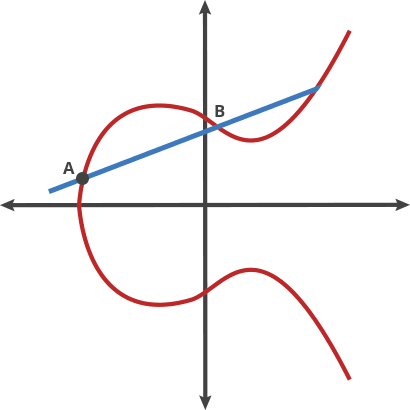We can call this billiards move on two points "dot." Any two points on a curve can be dotted together to get a new point.

A dot B = C

We can also string moves together to "dot" a point with itself over and over.

A dot A = B

A dot B = C

A dot C = D

...

It turns out that if you have two points, an initial point "dotted" with itself n times to arrive at a final point, finding out n when you only know the final point and the first point is hard. To continue our bizzaro billiards metaphor, imagine one person plays our game alone in a room for a random period of time. It is easy for him to hit the ball over and over following the rules described above. If someone walks into the room later and sees where the ball has ended up, even if they know all the rules of the game and where the ball started, they cannot determine the number of times the ball was struck to get there without running through the whole game again until the ball gets to the same point. Easy to do, hard to undo: this is the basis for a very good Trapdoor Function.

### Let's get weird

This simplified curve above is great to look at and explain the general concept of elliptic curves, but it doesn't represent what the curves used for cryptography look like.

For this, we have to restrict ourselves to numbers in a fixed range, like in RSA. Rather than allow any value for the points on the curve, we restrict ourselves to whole numbers in a fixed range. When computing the formula for the elliptic curve (y2 = x3 + ax + b), we use the same trick of rolling over numbers when we hit the maximum. If we pick the maximum to be a prime number, the elliptic curve is called a prime curve and has excellent cryptographic properties.

Here's an example of a curve (y2 = x3 - x + 1) plotted for all numbers: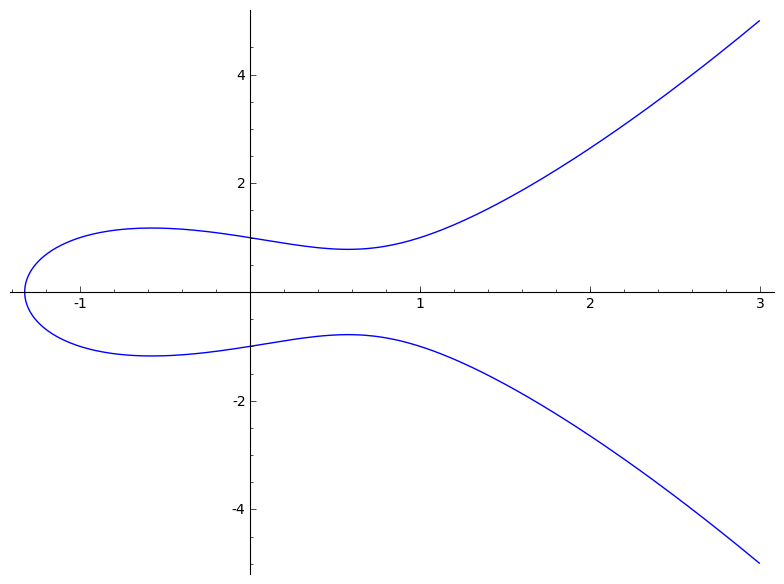Here's the plot of the same curve with only the whole number points represented with a maximum of 97: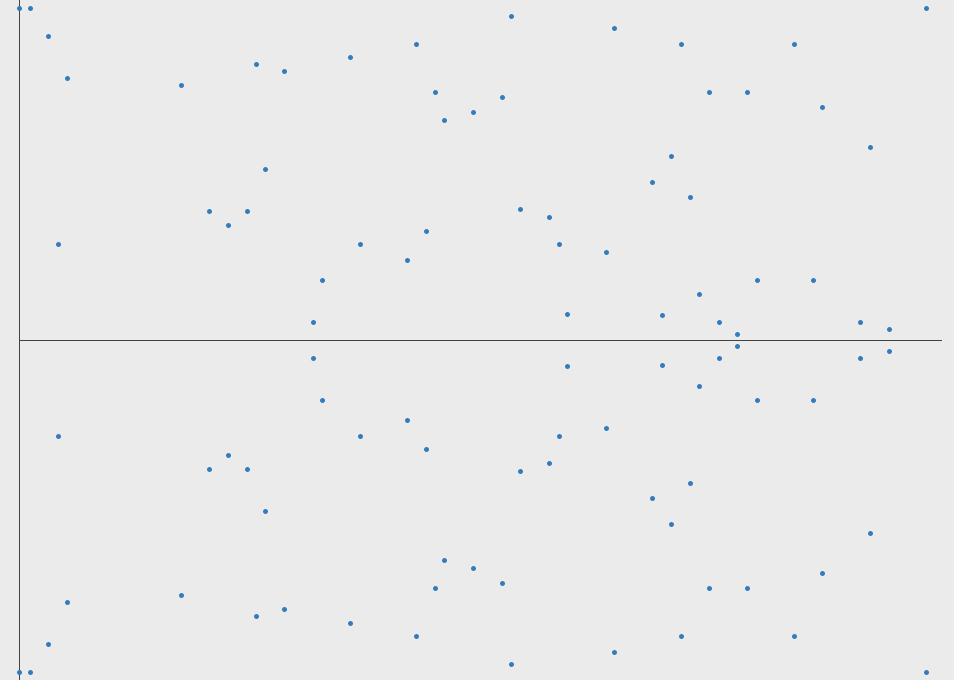This hardly looks like a curve in the traditional sense, but it is. It's like the original curve was wrapped around at the edges and only the parts of the curve that hit whole number coordinates are colored in. You can even still see the horizontal symmetry.

In fact, you can still play the billiards game on this curve and dot points together. The equation for a line on the curve still has the same properties. Moreover, the dot operation can be efficiently computed. You can visualize the line between two points as a line that wraps around at the borders until it hits a point. It's as if in our bizarro billiards game, when a ball hits the edge of the board (the max) then it is magically transported to the opposite side of the table and continues on its path until reaching a point, kind of like the game Asteroids.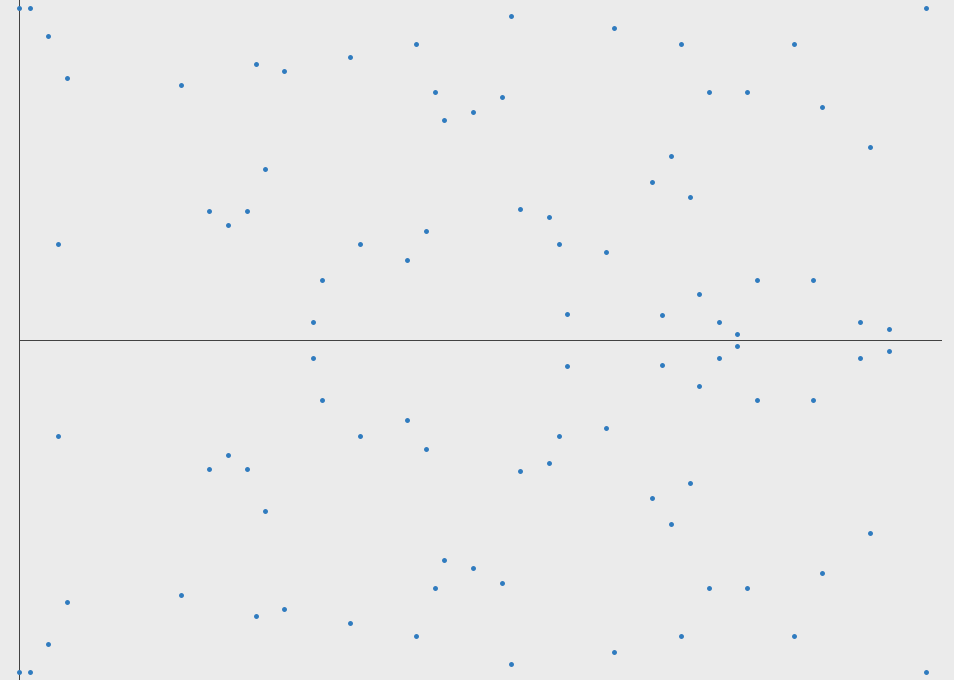With this new curve representation, you can take messages and represent them as points on the curve. You could imagine taking a message and setting it as the x coordinate, and solving for y to get a point on the curve. It is slightly more complicated than this in practice, but this is the general idea.

You get the points

(70,6), (76,48), -, (82,6), (69,22)

*There are no coordinates with 65 for the x value, this can be avoided in the real world

An elliptic curve cryptosystem can be defined by picking a prime number as a maximum, a curve equation and a public point on the curve. A private key is a number priv, and a public key is the public point dotted with itself priv times. Computing the private key from the public key in this kind of cryptosystem is called the elliptic curve discrete logarithm function. This turns out to be the Trapdoor Function we were looking for.

### What does it all mean?

The elliptic curve discrete logarithm is the hard problem underpinning elliptic curve cryptography. Despite almost three decades of research, mathematicians still haven't found an algorithm to solve this problem that improves upon the naive approach. In other words, unlike with factoring, based on currently understood mathematics there doesn't appear to be a shortcut that is narrowing the gap in a Trapdoor Function based around this problem. This means that for numbers of the same size, solving elliptic curve discrete logarithms is significantly harder than factoring. Since a more computationally intensive hard problem means a stronger cryptographic system, it follows that elliptic curve cryptosystems are harder to break than RSA and Diffie-Hellman.

To visualize how much harder it is to break, Lenstra recently introduced the concept of "Global Security." You can compute how much energy is needed to break a cryptographic algorithm, and compare that with how much water that energy could boil. This is a kind of cryptographic carbon footprint. By this measure, breaking a 228-bit RSA key requires less energy to than it takes to boil a teaspoon of water. Comparatively, breaking a 228-bit elliptic curve key requires enough energy to boil all the water on earth. For this level of security with RSA, you'd need a key with 2,380-bits.

With ECC, you can use smaller keys to get the same levels of security. Small keys are important, especially in a world where more and more cryptography is done on less powerful devices like mobile phones. While multiplying two prime numbers together is easier than factoring the product into its component parts, when the prime numbers start to get very long even just the multiplication step can take some time on a low powered device. While you could likely continue to keep RSA secure by increasing the key length that comes with a cost of slower cryptographic performance on the client. ECC appears to offer a better tradeoff: high security with short, fast keys.

### Elliptic curves in action

After a slow start, elliptic curve based algorithms are gaining popularity and the pace of adoption is accelerating. Elliptic curve cryptography is now used in a wide variety of applications: the U.S. government uses it to protect internal communications, the Tor project uses it to help assure anonymity, it is the mechanism used to prove ownership of bitcoins, it provides signatures in Apple's iMessage service, it is used to encrypt DNS information with DNSCurve, and it is the preferred method for authentication for secure web browsing over SSL/TLS. CloudFlare uses elliptic curve cryptography to provide perfect forward secrecy which is essential for online privacy. First generation cryptographic algorithms like RSA and Diffie-Hellman are still the norm in most arenas, but elliptic curve cryptography is quickly becoming the go-to solution for privacy and security online.

If you are accessing the HTTPS version of this blog (https://blog.cloudflare.com) from a recent enough version of Chrome or Firefox, your browser is using elliptic curve cryptography. You can check this yourself. In Chrome, you can click on the lock in the address bar and go to the connection tab to see which cryptographic algorithms were used in establishing the secure connection. Clicking on the lock in the Chrome 30 should show the following image.The relevant portions of this text to this discussion is ECDHE_RSA. ECDHE stands for Elliptic Curve Diffie Hellman Ephemeral and is a key exchange mechanism based on elliptic curves. This algorithm is used by CloudFlare to provide perfect forward secrecy in SSL. The RSA component means that RSA is used to prove the identity of the server.

We use RSA because CloudFlare's SSL certificate is bound to an RSA key pair. Modern browsers also support certificates based on elliptic curves. If CloudFlare's SSL certificate was an elliptic curve certificate this part of the page would state ECDHE_ECDSA. The proof of the identity of the server would be done using ECDSA, the Elliptic Curve Digital Signature Algorithm.

CloudFlare's ECC curve for ECDHE (This is the same curve used by Google.com):

```max: 115792089210356248762697446949407573530086143415290314195533631308867097853951
curve: y² = x³ + ax + b
a = 115792089210356248762697446949407573530086143415290314195533631308867097853948
b = 41058363725152142129326129780047268409114441015993725554835256314039467401291
```

The performance improvement of ECDSA over RSA is dramatic. Even with an older version of OpenSSL that does not have assembly-optimized elliptic curve code, an ECDSA signature with a 256-bit key is over 20x faster than an RSA signature with a 2,048-bit key.

On a MacBook Pro with OpenSSL 0.9.8, the "speed" benchmark returns:

```Doing 256 bit sign ecdsa's for 10s: 42874 256 bit ECDSA signs in 9.99s
Doing 2048 bit private rsa's for 10s: 1864 2048 bit private RSA's in 9.99s
```

That's 23x as many signatures using ECDSA as RSA.

CloudFlare is constantly looking to improve SSL performance. Just this week, CloudFlare started using an assembly-optimized version of ECC that more than doubles the speed of ECDHE. Using elliptic curve cryptography saves time, power and computational resources for both the server and the browser helping us make the web both faster and more secure.

### The downside

It is not all roses in the world of elliptic curves, there have been some questions and uncertainties that have held them back from being fully embraced by everyone in the industry.

One point that has been in the news recently is the Dual Elliptic Curve Deterministic Random Bit Generator (Dual_EC_DRBG). This is a random number generator standardized by the National Institute of Standards and Technology (NIST), and promoted by the NSA. Dual_EC_DRBG generates random-looking numbers using the mathematics of elliptic curves. The algorithm itself involves taking points on a curve and repeatedly performing an elliptic curve "dot" operation. After publication it was reported that it could have been designed with a backdoor, meaning that the sequence of numbers returned could be fully predicted by someone with the right secret number. Recently, the company RSA recalled several of their products because this random number generator was set as the default PRNG for their line of security products. Whether or not this random number generator was written with a backdoor or not does not change the strength of the elliptic curve technology itself, but it does raise questions about the standardization process for elliptic curves. As we've written about before, it's also part of the reason that attention should be spent to ensuring that your system is using adequately random numbers. In a future blog post, we will go into how a backdoor could be snuck into the specification of this algorithm.

Some of the more skeptical cryptographers in the world now have a general distrust for NIST itself and the standards it has published that were supported by the NSA. Almost all of the widely implemented elliptic curves fall into this category. There are no known attacks on these special curves, chosen for their efficient arithmetic, however bad curves do exist and some feel it is better to be safe than sorry. There has been progress in developing curves with efficient arithmetic outside of NIST, including curve 25519 created by Daniel Bernstein (djb) and more recently computed curves by Paulo Baretto and collaborators, though widespread adoption of these curves are several years away. Until these non-traditional curves are implemented by browsers, they won't be able to be used for securing cryptographic transport on the web.

Another uncertainty about elliptic curve cryptography is related to patents. There are over 130 patents that cover specific uses of elliptic curves owned by BlackBerry (through their 2009 acquisition of Certicom). Many of these patents were licensed for use by private organizations and even the NSA. This has given some developers pause over whether their implementations of ECC infringe upon this patent portfolio. In 2007, Certicom filed suit against Sony for some uses of elliptic curves, however that lawsuit was dismissed in 2009. There are now many implementations of elliptic curve cryptography that are thought to not infringe upon these patents and are in wide use.

The ECDSA digital signature has a drawback compared to RSA in that it requires a good source of entropy. Without proper randomness, the private key could be revealed. A flaw in the random number generator on Android allowed hackers to find the ECDSA private key used to protect the bitcoin wallets of several people in early 2013. Sony's Playstation implementation of ECDSA had a similar vulnerability. A good source of random numbers is needed on the machine making the signatures. Dual_EC_DRBG is not recommended.

Even with the above cautions, the advantages of elliptic curve cryptography over traditional RSA are widely accepted. Many experts are concerned that the mathematical algorithms behind RSA and Diffie-Hellman could be broken within 5 years, leaving ECC as the only reasonable alternative.

Elliptic curves are supported by all modern browsers, and most certification authorities offer elliptic curve certificates. Every SSL connection for a CloudFlare protected site will default to ECC on a modern browser. Soon, CloudFlare will allow customers to upload their own elliptic curve certificates. This will allow ECC to be used for identity verification as well as securing the underlying message, speeding up HTTPS sessions across the board. More on this when the feature becomes available.

We protect entire corporate networks, help customers build Internet-scale applications efficiently, accelerate any website or Internet application, ward off DDoS attacks, keep hackers at bay, and can help you on your journey to Zero Trust.

Visit 1.1.1.1 from any device to get started with our free app that makes your Internet faster and safer.

To learn more about our mission to help build a better Internet, start here. If you're looking for a new career direction, check out our open positions.

HTTPS Elliptic Curves Crypto Security